Vibroengineering PROCEDIA

Published: 25 August 2022

# Applications of bispectrum analysis to inspection of fatigue damage in quay cranes

Li Cui1
Maosen Cao2
Hao Zhang3
Shuigen Hu4
Jie Wang5
1, 2, 3, 4Anhui Provincial International Joint Research Center of Data Diagnosis and Smart Maintenance on Bridge Structures, Chuzhou University, Anhui, People’s Republic of China
5Intelligent Transportation and Intelligent Construction Engineering Research Center, Jiangsu Dongjiao Intelligent Control Technology Group Co., Nanjing, China
1, 2, 3, 4College of Civil and Architecture Engineering, Chuzhou University, Anhui, People’s Republic of China
Corresponding Author:
Maosen Cao
Editor's Pick
Views 19

#### Abstract

The quay crane is a special mechanical equipment which can lift the container vertically and horizontally through intermittent circulation movement. Fatigue crack is one of the main kinds of damage in quay cranes. The generation and development of fatigue crack are invisible, which makes the identification of fatigue cracks a great challenge. From the dynamic point of view, fatigue cracks have nonlinear dynamic characteristics beyond the linear dynamic category. The traditional damage detection methods based on linear hypothesis are difficult to effectively recognize the nonlinear dynamic characteristics of fatigue cracks, so they cannot be effectively used for structural fatigue crack detection. In order to overcome the limitations of the existing linear damage detection methods, bispectrum analysis, a nonlinear dynamic analysis method, is introduced in this thesis. By drawing on the use of bispectrum analysis to describe the nonlinear dynamic characteristics of rotating structures, a fatigue crack detection method based on nonlinear dynamic characteristics of quay cranes is developed.

## 1. Introduction

Quay Cranes are in a very important and unique position in the increasingly fierce international trade and port competition. Quay Cranes are typical welded metal structures. In addition, when the quay crane is working, the trolley is constantly started and braked, or the suspended load is shuttling between the front and rear main beams at high speed, resulting in alternating stress in the structure and causing dislocation movement accumulation and fatigue cracks. However, the current main health monitoring methods of quay cranes are still based on the visual inspection of experienced personnel and magnetic particle inspection, which obviously cannot meet the huge demand of quay cranes .

## 2. Bispectrum analysis method

It is assumed that the mean value of real random signal $\left\{x\left(n\right)\right\}$ is zero and $k$-order is stationary. If the $k$-order cumulant ${c}_{kx}\left({\tau }_{1},{\tau }_{2},\dots ,{\tau }_{k-1}\right)$ of $\left\{x\left(n\right)\right\}$ exists, the $k$-order spectrum is defined as the ($k-1$)-dimensional Fourier transform of ${c}_{kx}\left({\tau }_{1},{\tau }_{2},\dots ,{\tau }_{k-1}\right)$:

1
${S}_{kx}\left({\omega }_{1},{\omega }_{2},\dots ,{\omega }_{k-1}\right)={\sum }_{{\tau }_{1}=-\mathrm{\infty }}^{\mathrm{\infty }}\dots {\sum }_{{\tau }_{k-1}=-\mathrm{\infty }}^{\mathrm{\infty }}{\tau }_{1},{\tau }_{2},\dots ,{\tau }_{k-1}\bullet \mathrm{e}\mathrm{x}\mathrm{p}\left[-i{\sum }_{j=1}^{k-1}{\omega }_{j}{\tau }_{j}\right].$

When $k=$ 3, the third-order spectrum ${S}_{3x}$ is also called bispectrum, expressed in ${B}_{x}\left({\omega }_{1},{\omega }_{2}\right)$.

The estimated bispectrum[5-6]can be expressed as:

2
$B\left({\omega }_{1},{\omega }_{2}\right)=X\left({\omega }_{1}\right)X\left({\omega }_{2}\right){X}^{\mathrm{*}}\left({\omega }_{1}+{\omega }_{2}\right),$

where $X\left(k\right)={\sum }_{n=0}^{N-1}x\left(n\right){e}^{-i\frac{2\pi }{N}nk}\left(0\le k\le N-1\right)$ is the Fourier transform determined by the sequence of principal values of $\left\{x\left(n\right)\right\}$; $\mathrm{*}$ means complex conjugate.

${\omega }_{1}$ and ${\omega }_{2}$ in $B\left({\omega }_{1},{\omega }_{2}\right)$ are two mutually independent frequencies, which can represent non minimum phase information. $B\left({\omega }_{1},{\omega }_{2}\right)$ is a double periodic function with the same period. The period is 2$\pi$, and satisfies:

3
$B\left({\omega }_{1},{\omega }_{2}\right)={B}^{\mathrm{*}}\left(-{\omega }_{1},-{\omega }_{2}\right)=B\left(-{\omega }_{1}-{\omega }_{2},{\omega }_{2}\right)==B\left(-{\omega }_{1}-{\omega }_{2},{\omega }_{1}\right)=B\left({\omega }_{2},{\omega }_{1}\right)={B}^{\mathrm{*}}\left(-{\omega }_{2},-{\omega }_{1}\right)=B\left({\omega }_{2},-{\omega }_{1}-{\omega }_{2}\right)=B\left({\omega }_{1},-{\omega }_{1}-{\omega }_{2}\right).$

There are six symmetry lines which are ${\omega }_{1}={\omega }_{2}$, $2{\omega }_{1}=-{\omega }_{2}$, $-{\omega }_{1}=2{\omega }_{2}$, ${\omega }_{1}=-{\omega }_{2}$, ${\omega }_{1}=0$ and ${\omega }_{2}=0$ in $B\left({\omega }_{1},{\omega }_{2}\right)$. Since the bispectrum satisfies such high symmetry, all the bispectrum can pass through the symmetric region ${\omega }_{2}\ge 0$, ${\omega }_{1}\ge {\omega }_{2}$, ${\omega }_{1}+{\omega }_{2}\le \pi$.

## 3. The finite element simulation

In this paper, an 80 t-65 m quay crane is taken as the prototype to build the finite element model. The relevant parameters are shown in Table 1. The material of the quay crane is Q345 steel, and the material property is: elastic modulus 2.06×1011 Pa, Poisson's ratio $\upsilon$ = 0.3, density $\rho$ = 7.85×103 kg/m3. In this paper, the quay crane is set in the working state, that is, the crane is completely locked and in the windless environment. Therefore, the front girder can be regarded as a complex cantilever beam model, and fixed constraints will be set at the bolt holes on the land side. This is to simplify the model and calculation.

Table 1Main parameters of the model

 Front extension (m) Back extension (m) Crane gauge (m) Trolley gauge (m) Base distance (m) Load capacity (t) 65 30.5 35 6.3 20.5 Sling Cliver 65 80

A 50 %- degree breathing crack is set at five positions as shown in Fig. 1. respectively. A simple harmonic excitation $F\mathrm{sin}\omega t$ is set on the upper surface of the free end near the sea side. 50000 N, $\omega ={f}_{1}/2$ (${f}_{1}=$ 0.353 Hz is the first-order natural frequency of the front girder with a breathing crack). The acceleration response of the structure is extracted at the lower surface of the free end near the sea side, and bispectrum analysis is performed. The results are shown in Fig. 2.

When the breathing crack transits from the fixed end near the land side to the free end near the sea side, the second harmonic in the bispectrum gradually changes from obvious to not obvious, but the amplitude of the second harmonic is the smallest in the middle section of the front girder; When the crack is close to the fixed end on the land side and the free end on the sea side, the amplitude of the second harmonic is larger. However, it should be noted that this change rule is not invariable, and may generally change due to the difference between the position where the excitation is applied and the position where the dynamic response is extracted.

Fig. 1Diagram of damage position on the front girder of the quay bridge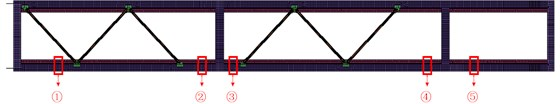Fig. 2Three-dimensional bispectrum when the damage is at different position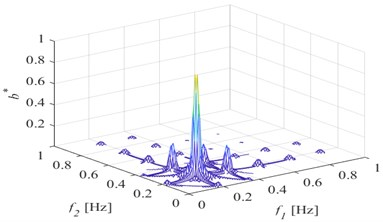a) Position 1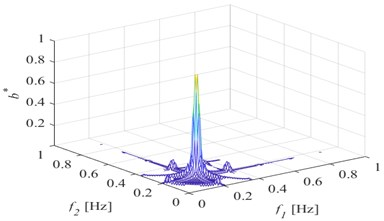b) Position 2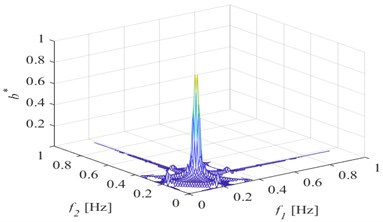c) Position 3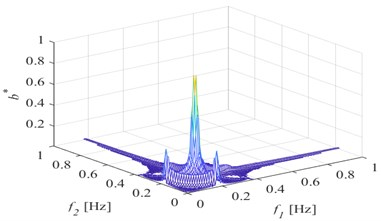d) Position 4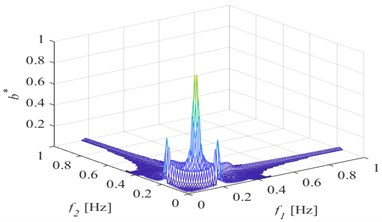e) Position 5

A breathing crack is set at position 1 near the fixed end, and the crack depth $p$ is set as 10 %, 20 %, 30 %, 40 %, and 50 %, respectively to study the change of the bispectral nonlinear dynamic characteristics under different damage degrees. A simple harmonic excitation $F\mathrm{sin}\omega t$ is set on the upper surface of the free end near the sea side, where the excitation size $F$ is set to 50 000 N and the excitation frequency $\omega$ is set to ${f}_{1}/2$. The acceleration response of the structure is extracted at the lower surface of the free end near the sea side, and bispectrum analysis is performed. The results are shown in Fig. 3.

Fig. 3Bispectrum when a) p= 10 %; b) p= 20 %; c) p= 30 %; d) p= 40 %; e) p= 50 %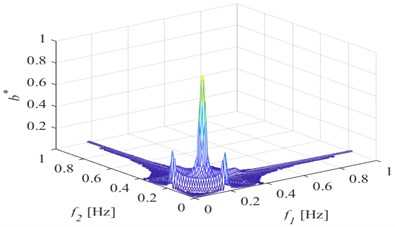a)10 %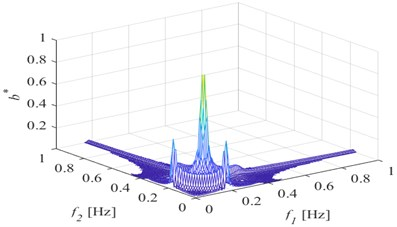b)20 %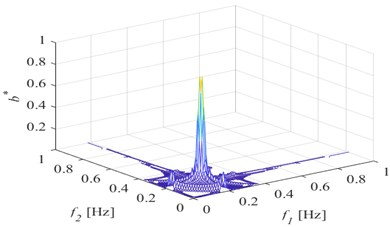c)30 %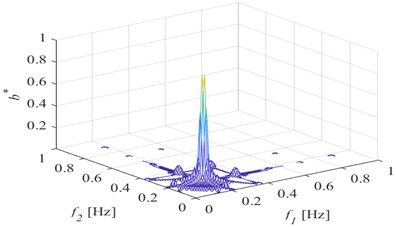d)40 %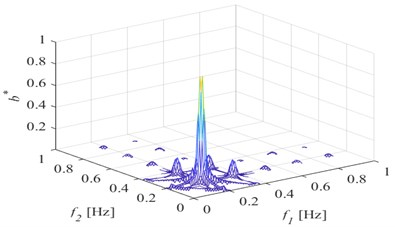e)$\mathrm{p}=$ 50 %

Fig. 4Variation trend of the second harmonic amplitude with crack depth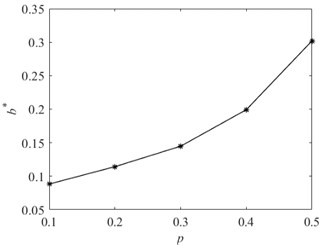Fig. 3 shows that the second harmonic component of the bispectrum becomes more and more obvious with the deepening of the damage degree, and the amplitude is also larger and larger. Among them, when the damage degree is 10 % and 20 %, the second harmonic component can be observed, but it is not obvious compared with the case of 50 % damage depth, which may be related to the monitoring position and normalization processing method. Extract the second harmonic amplitude under different crack depths, and the change is shown in Fig. 4. It can be seen from the figure that the amplitude of the second harmonic of the bispectrum monotonically increases with the crack depth. Therefore, the second harmonic component of the bispectrum can be used as a characteristic index to identify the fatigue damage degree.

## 4. Conclusions

In this paper, the bispectrum dynamic analysis is carried out on the quay crane which is under working condition. The results show that bispectrum analysis can extract the nonlinear dynamic characteristics of the crane structure with fatigue cracks. The bispectral nonlinear dynamic characteristics are positively correlated with the depth of fatigue crack in the structure. The bispectral nonlinear dynamic characteristics no longer show a single change law with the change of crack position. The research results show that the proposed fatigue crack detection method based on bispectrum analysis can capture the nonlinear dynamic characteristics of fatigue cracks in the structure of quay cranes under different working conditions, and provides a new technology for fatigue crack identification of quay cranes. It must be noted that, bispectrum analysis method has shown unprecedented advantages in the fault detection of structure components, especially rotating components, but it is rarely used in the damage detection of large and complex structures. Therefore, this paper only proposes new possibilities for the fatigue crack detection of quay cranes. However, if this method is really to be used in the actual structure monitoring, it must be further studied.

#### Acknowledgements

This work is supported by Anhui provincial international joint research center of data diagnosis and smart maintenance on bridge structures (No. 2021AHGHYB05), the University scientific research project of Anhui Provincial Department of Education (Nos. KJ2021A1097 and KJ2021A1098), the Key R&D Project of Anhui Science and Technology Department (202004b11020026), and Nanjing International Joint Research and Development Program (No. 202112003).